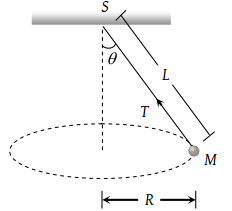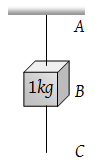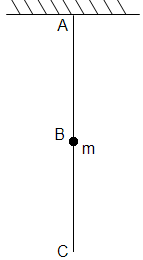A bucket full of water is revolved in vertical circle of radius 2m. What should be the maximum time-period of revolution so that the water doesn't fall off the bucket

(1) 1 sec

(2) 2 sec

(3) 3 sec

(4) 4 sec

Concept Questions :-

Uniform circular motion
High Yielding Test Series + Question Bank - NEET 2020

Difficulty Level:

A tube of length L is filled completely with an incompressible liquid of mass M and closed at both the ends. The tube is then rotated in a horizontal plane about one of its ends with a uniform angular velocity ω. The force exerted by the liquid at the other end is

(1) $\frac{ML{\omega }^{2}}{2}$

(2) $ML{\omega }^{2}$

(3) $\frac{ML{\omega }^{2}}{4}$

(4) $\frac{M{L}^{2}{\omega }^{2}}{2}$

High Yielding Test Series + Question Bank - NEET 2020

Difficulty Level:

A string of length L is fixed at one end and carries a mass M at the other end. The string makes 2/π revolutions per second around the vertical axis through the fixed end as shown in the figure, then tension in the string is(1) ML

(2) 2 ML

(3) 4 ML

(4) 16 ML

High Yielding Test Series + Question Bank - NEET 2020

Difficulty Level:

A long horizontal rod has a bead which can slide along its length, and initially placed at a distance L from one end A of the rod. The rod is set in angular motion about A with constant angular acceleration $\alpha$. If the coefficient of friction between the rod and the bead is μ, and gravity is neglected, then the time after which the bead starts slipping is

(1) $\sqrt{\frac{\mu }{\alpha }}$

(2) $\frac{\mu }{\sqrt{\alpha }}$

(3) $\frac{1}{\sqrt{\mu \alpha }}$

(4) Infinitesimal

Concept Questions :-

Uniform circular motion
High Yielding Test Series + Question Bank - NEET 2020

Difficulty Level:

A particle is moving with a constant speed along a straight line path. A force is not required to

(1) Increase its speed

(2) Decrease the momentum

(3) Change the direction

(4) Keep it moving with uniform velocity

Concept Questions :-

Newton laws
High Yielding Test Series + Question Bank - NEET 2020

Difficulty Level:

A mass of 1 kg is suspended by a string A. Another string C is connected to its lower end (see figure). If a sudden jerk is given to C, then(1) The portion AB of the string will break

(2) The portion BC of the string will break

(3) None of the strings will break

(4) The mass will start rotating

Concept Questions :-

Newton laws
High Yielding Test Series + Question Bank - NEET 2020

Difficulty Level:

In the  Question, if the string C is stretched slowly, then(1) The portion AB of the string will break

(2) The portion BC of the string will break

(3) None of the strings will break

(4) None of the above

Concept Questions :-

Newton laws
High Yielding Test Series + Question Bank - NEET 2020

Difficulty Level:

Newton's second law gives the measure of

(1) Acceleration

(2) Force

(3) Momentum

(4) Angular momentum

Concept Questions :-

Newton laws
High Yielding Test Series + Question Bank - NEET 2020

Difficulty Level:

A coin is dropped in a lift. It takes time t1 to reach the floor when lift is stationary. It takes time t2 when lift is moving up with constant acceleration. Then

(1) t1 > t2

(2) t2 > t1

(3) t1 = t2

(4) t1 >> t2

Concept Questions :-

Application of laws
High Yielding Test Series + Question Bank - NEET 2020

Difficulty Level:

If the tension in the cable of 1000 kg elevator is 1000 kg weight, the elevator

(1) Is accelerating upwards

(2) Is accelerating downwards

(3) May be at rest or accelerating

(4) May be at rest or in uniform motion

Concept Questions :-

Newton laws